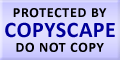Submit Assignment From Here
Name
:
Email
:
Phone
:
Country
:
Willing to pay US\$
:
Subject
:
Topic
:
Levels
:
:Hours
:

# Nonparametric Tests Homework Help

Sometimes the t – tests are not able to give the user an appropriate solution they get violated. The data does not have any interval or is consistent in nature. In such case we can use the nonparametric test approach. Nonparametric test approach is used occasionally. There are nonparametric approaches which do not require any particular form of distribution. Common nonparametric tests are not used for real values of observation. These are also called distribution free tests. Nonparametric test approach does not require assumptions related to normality of variance or homogeneity of variance. In nonparametric test approach we make a comparison between the median and not between the means. Chi – square is considered a nonparametric test, Spearman correlation is also nonparametric test. The table below shows the comparison between the normal test and rank test which is as follows:

Basis                                          Normal test                                Rank test
One sample                                one sample t – test                     Wilcoxon signed rank test
Matched pairs                             apply one – sample test               Apply one – sample test to
To differences within the pairs       difference within the pairs.
Two independent samples            two samples t – test                    Wilcoxon rank sum test
Many independent samples          one way AVONA F – test              Kruskal – Wallis testUS